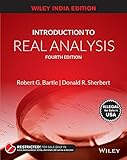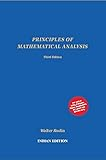# CSIR-NET: Analysis/Topology Quiz 1

Let A be a closed subset of . Then A is

The closure of interior of A
Hint: Is {1, 3, 5} closed? What can you say about the closure of interior of this set?

A countable set
Hint: Is closed? Is it countable? You can try guess the answer again!

A compact set
Hint: Is closed? Is it compact? You can try guess the answer again!

Not open
is a connected space. And by the definition of connected space, only open and closed sets are . Watch more solutions in this video.

CSIR-NET December 2015, Part B Question.

How should I analyze this question when I am preparing for CSIR-NET?

1. Mark all mathematical terms here. Read their definitions.
2. Try construct examples for each terms, try apply it in options.
3. This question is interesting because it talks about a lot of mathematical terms, but the keyword is nowhere mentioned in question – connected spaces. Read and analyse that definition, too.
4. Make a note of all your observations, examples that you found, in your NET preparation diary. For example, you should have asked questions like:
1. Why did they say closure of interior of A, and not interior of closure of A? Will there be any difference?
2. How will countable closed sets look like?
3. Can there be countable open sets?
4. Are there any other sets which are connected, like$\mathbb R$?
5. Can I characterize compact sets which are not closed in$\mathbb R$?

Once you are done, try watching the following video. Is there any other ways to solve the same question?

Consider buying one of the following suggested books, if you do not have it yet.

## Recommended Books for Real AnalysisIntroduction to Real Analysis by Bartle and Sherbert (4e)Principles of Mathematical Analysis by Rudin